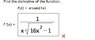# Inverse Trig Function: Find Derivative of the Function

chapsticks

## Homework Statement

find the derivative of the function
f(x)=arcsec(4x)

## Homework Equations

I think this is a Relevant equations.

d/dx[arcsecu]=u'/(|u|(√u2-1)

## The Attempt at a Solution

f'(x)=4/(|4|(√42-1)
=1/√15

I keep getting wrong in my online homework why?Homework Helper

## Homework Statement

find the derivative of the function
f(x)=arcsec(4x)

## Homework Equations

I think this is a Relevant equations.

d/dx[arcsecu]=u'/(|u|(√u2-1)

## The Attempt at a Solution

f'(x)=4/(|4|(√42-1)
=1/√15

I keep getting wrong in my online homework why?What happened to the x??

chapsticks
is it 4/(|4x|(√4x2-1))

I keep getting it wrong

Homework Helper
What is u in your original integral? What is $u^2$?

Homework Helper
is it 4/(|4x|(√4x2-1))

I keep getting it wrong

That's sort of close. But look up the formula again. Isn't the square root part $\sqrt(u^2-1)$ instead of what you have? And when you write something like 4x^2 it's not clear whether you mean (4x)^2 or 4*(x^2). Which do you mean?

chapsticks
I mean this one (4x)^2

Homework Helper
I mean this one (4x)^2

Ok, then keep writing it like that. And what about my other question?

chapsticks

f'(x)=arcsec4x+ 4/(4x(√(16x)2-1)

chapsticks
I did this one in my homework online and it keeps saying I'm wrong

#### Attachments

•asd.jpg
6.1 KB · Views: 509
Homework Helper

f'(x)=arcsec4x+ 4/(4x(√(16x)2-1)

Stop changing things without giving any reason. Why did you put the arcsec4x in there? Why did you drop the absolute value on |4x|? (4x)^2 was right, (16x)^2 isn't. Why not?